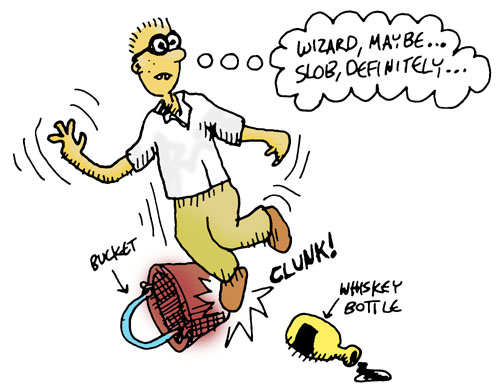## Finding Things

We still have one thing we need to describe: If there are any objects on the floor at the location we are standing in, we'll want to describe them as well. Let's first write a helper function that tells us whether an item is in a given place:

``````(defun item-there?
((loc (match-object location obj-loc)) (when (== loc obj-loc))
'true)
((_ _)
'false))
``````

This is very similar to one of our previous functions, having both pattern matching and a guard. Our function above is 2-arity, with the first argument being the location, and the second argument the expression for matching an `object` record.

That's the first function head pattern that `there?` has. The second function head pattern has two arguments, just like the first one, but in this case the function arguments are both the "don't care" variable: the underscore. This part of the function is saying, "If you've made it past the first pattern without matching, I don't care what your location is or what your record is: I'm going to return `false`. In other words, since there was no match, the queried item is not present.

Let's try out that function in the REPL. This will tell us if the first object in the list of game objects is in the living room:

``````> (item-there? 'living-room (car (state-objects state)))
true
> (item-there? 'attic (car (state-objects state)))
false
``````

Remember that `(state-objects state)` returns all game objects. Let's use `lists:filter` like we did before with the place descriptions:

``````(defun whats-here?
(((match-state player-location player-loc objects objs))
(lists:filter
(lambda (obj)
(item-there? player-loc obj))
objs)))
``````

Now let's try it out:

``````> (whats-here? state)
``````
``````(#(object whiskey-bottle living-room) #(object bucket living-room))
``````You know how, in the last section, we described a single exit and then a list of exits? We're going to do the same thing now. In fact, the functions are very similar. Here's the function for describing an item:

``````(defun describe-item
(((match-object name obj-name))
(++ "You see a " (atom_to_list obj-name) " on the ground.")))
``````
``````(defun add-newline
(('()) '())
((string) (++ string "\n")))
``````

That last function is to prevent a newline being printed when there are no items to describe. What it's saying is "if I get an argument that matches an empty list, I'll just return an empty list; if I get a non-empty list, I'll append a newline character."

Now let's use this function and our `whats-here?` function to describe all the items in the current room:

``````(defun describe-items (game-state)
(string:join
(lists:map
#'describe-item/1
(whats-here? game-state))
" ")))
``````

There's nothing new here -- you've seen all of this before. You're starting to get it, right? Let's try our latest function:

``````> (describe-items state)
``````
``````"You see a whiskey-bottle on the ground. You see a bucket on the ground.\n"
``````

We're doing a good job defining the puzzle pieces. Next we'll see how they start to fit together ...